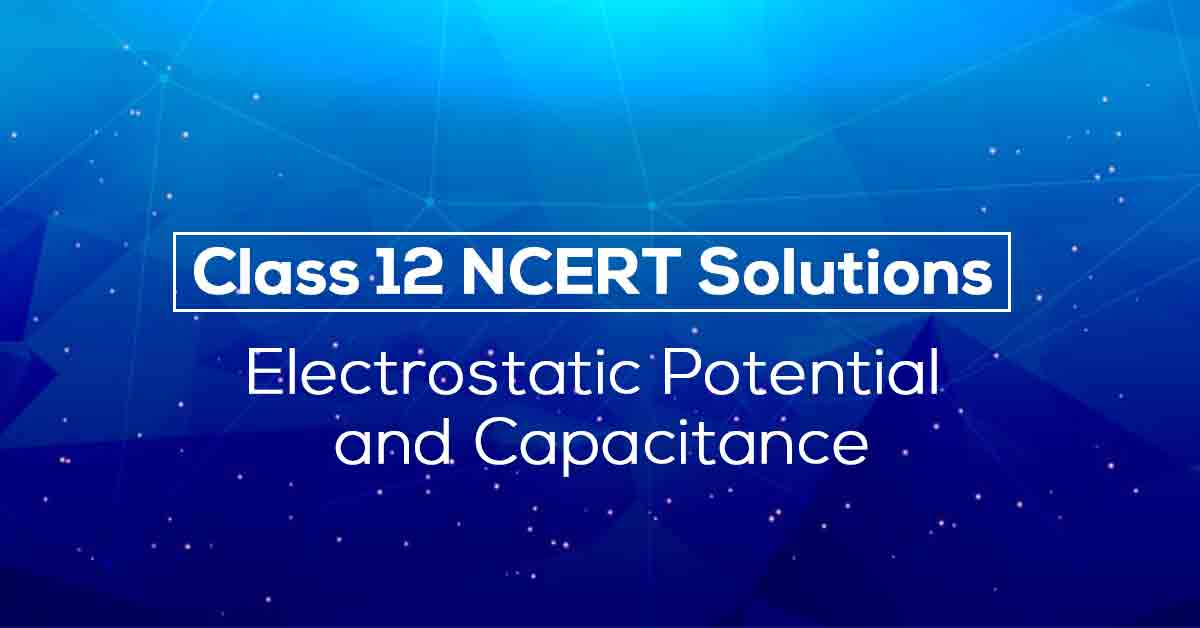Class 12th Physics Electrostatic Potential and Capacitance NCERT Solution CBSE 2023

# NCERT Solutions for Class 12 Physics Chapter 2## NCERT Solutions for Class 12 Physics Chapter 2 Electrostatic Potential and Capacitance – PDF Download

Vidyakul presents NCERT Solutions for Class 12 Physics Chapter 2 for students who want to learn fast and score more in the examination. These NCERT Solutions for Class 12 Physics Chapter 2 answers all the questions mentioned in Chapter - 2 Electrostatic Potential and Capacitance of NCERT Textbook. The NCERT Solutions for Class 12 Physics Chapter 2 pdf also contains notes which would help you understand the basic concepts in a simple yet effective way.

Electrostatic Potential and Capacitance is an essential chapter in class 12 Physics, both from the board’s perspective as well as competitive exam’s perspective. The NCERT Solutions for Class 12 Physics Chapter 2  PDF covers the entire CBSE Physics syllabus and solutions to in text questions as well. Thus students are advised to follow these solutions and notes as not only they will help them to achieve more marks, but could also be used as the answer key to complete an assignment or homework faster.

Given below is an overview of the topics covered in NCERT Solutions for Class 12 Physics Chapter 2 PDF:

• INTRODUCTION
• ELECTROSTATIC POTENTIAL
• POTENTIAL DUE TO A POINT CHARGE
• POTENTIAL DUE TO AN ELECTRIC DIPOLE
• POTENTIAL DUE TO A SYSTEM OF CHARGES
• EQUIPOTENTIAL SURFACES
• POTENTIAL ENERGY OF A SYSTEM OF CHARGES
• POTENTIAL ENERGY IN AN EXTERNAL FIELD
• ELECTROSTATICS OF CONDUCTORS
• DIELECTRICS AND POLARISATION
• CAPACITORS AND CAPACITANCE
• THE PARALLEL PLATE CAPACITOR
• EFFECT OF DIELECTRIC ON CAPACITANCE
• COMBINATION OF CAPACITORS
• ENERGY STORED IN A CAPACITOR
• VAN DE GRAAFF GENERATOR.

Electrostatic potential and Capacitance is about the electrostatic potential which means moving a unit positive charge to one point to other and the capacity of a conductor to store electric charge with suitable examples and diagrams. Know More about this in NCERT Solutions for Class 12 Physics Chapter 2 PDF.

Download the FREE PDF of NCERT Solutions for Class 12 Physics Chapter 2 - Electrostatic Potential and Capacitance and start your preparation with Vidyakul!